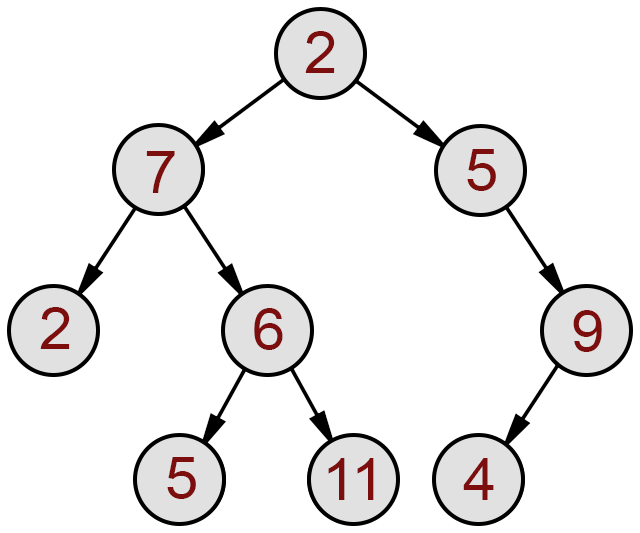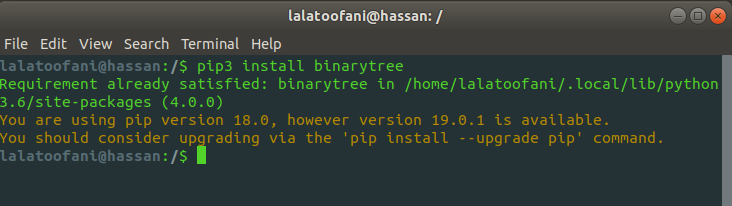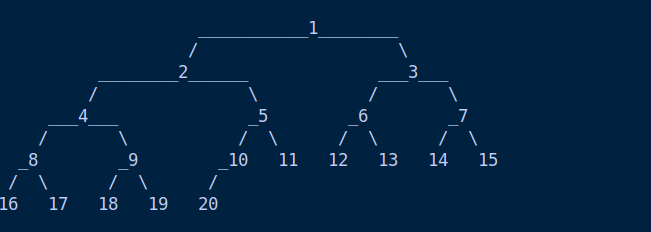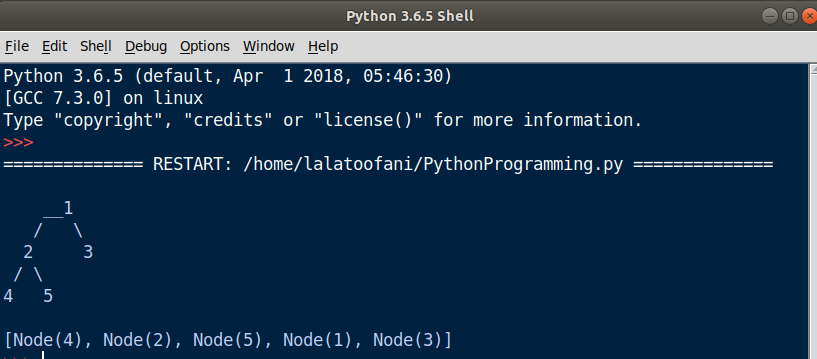## Tree Data Structure In Python

##### Python Trees: Learn Python Tree Data Structure in Detail with Real Examples
Written by |27-Apr-2019 | 0 Comments | 952 Views

The tree is another data structure that allows the data to reside in a hierarchical position. Each tree has a node, and each node has a leaf also called child. In python, there is no such data structure built-in. However, the open source community have contributed in developing tree data structures in python. It is not very efficient yet. Many modules have been developed, but the binary tree is one of the best to understand the basic concept of the tree which helps to understand graph data structure in the next lectures. The tree data structure look like this:The above-given image is of a binary tree. Here each circle is a node, and each node has further two leaf nodes which are nodes itself. The nodes cannot be more than 2 in a binary tree. Each node contains the data just like the element of the list include a value.

So how can we implement this in python?

To implement this in python, we have to use a module known as a binary tree. As it is not a built-in module, we first have to install it via command line as follows:In the above image shows that I have already downloaded it on my computer, so it satisfied the requirement. To run the code in python following method is used:

`Code`
` `
`from binarytree import Node`

`root = Node (3)`
`root.left = Node (4)`
`root.right = Node (9)`

`root.right.left = Node (16)`

`print(root)`

From the above-given code, we can see that we first assign a node having value 3. Then we assign left node leaf having value 4 and right leaf node having value 9. further we assign right leaf node a child leaf having value 16. When we print the root following output is given as:

 3_  /    4     _9      /     16

The output is given a tree. However, this method is a bit lengthy. We have to assign each node by hand which is a lengthy process. We can directly convert the list into a tree data structure by the following method as follows:

`Code`

`from binarytree import build`
`values = [1,2,3,4,5,6,7,8,9,10,11,12,13,14,15,16,17,18,19,20]`
`root = build(values)`
`print(root)`The output of the above code is as follows. As we can see the trend, the tree is built in left to right fashion when converted from the list.

Tree Traversal:

Unlike arrays and list in which we can iterate over those data structure in only one way from left to right. In a tree, we can iterate the tree in 3 different ways. This is known as tree traversal. There are three types of tree traversal. Following are the name of tree traversal:

• In order
• Pre-order
• Post order

## In order tree traversal (left, root, right):

• First visits the left subtree
• Than visit root
• In Last visit right subtree

`Code`

`from binarytree import build`
`values = [1,2,3,4,5]`
`root = build(values)`
`print(root)`
`print(root.inorder)`Pre-order (Root, Left, Right):

• First, visit the root
• then the left leaf node
• in last right leaf node
`Code`

`from binarytree import build`
`values = [1,2,3,4,5]`
`root = build(values)`
`print(root)`
`print(root.preorder)`

Post order (left, right, root):

• First, visit the left leaf node
• then the right leaf node
• in last visit root
`Code`

`from binarytree import build`
`values = [1,2,3,4,5]`
`root = build(values)`
`print(root)`
`print(root.postorder)`